In this tutorial, we will learn to:

• Represent online conversations in array structures.
• Model online forums using the model Gomez 2013.
• Check a model.
• Extend the current code to new (better?) models.

# Preliminaries (10 mins)

Open R Studio and install some packages we will need:

install.packages("igraph")   # Classic R package for graphs
install.packages("ggplot2")  # Classic R package for plots
install.packages("tidyr")    # Classic R package to manipulate data
install.packages("dplyr")    # Classic R package to manipulate data
install.packages("dfoptim")  # R package with Nelder-Mead optimizer

• (if you have git): git clone https://github.com/alumbreras/generative-discussion-threads.git

Open R Studio, and from there open any file from our package. For instance, open this file, located at

analysis/Tutorial ASONAM/notebookR_asonam.Rmd 

and then tell R to make this directory its working directory:

Session -> Set Working Directory -> To Source File Location

Finally, tell R to load the package (a fake install).

devtools::load_all() # load the functions of the package
Loading genthreads

Attaching package: ‘dplyr’

The following objects are masked from ‘package:stats’:

filter, lag

The following objects are masked from ‘package:base’:

intersect, setdiff, setequal, union

data.table 1.10.4.3
The fastest way to learn (by data.table authors): https://www.datacamp.com/courses/data-analysis-the-data-table-way
Documentation: ?data.table, example(data.table) and browseVignettes("data.table")
Release notes, videos and slides: http://r-datatable.com

Attaching package: ‘data.table’

The following objects are masked from ‘package:dplyr’:

between, first, last

Attaching package: ‘igraph’

The following objects are masked from ‘package:dplyr’:

as_data_frame, groups, union

The following objects are masked from ‘package:stats’:

decompose, spectrum

The following object is masked from ‘package:base’:

union

Attaching package: ‘gridExtra’

The following object is masked from ‘package:dplyr’:

combine

Now we are ready to go!

# Input data (5 mins)

### Basic data structures

We will first learn how to represent the structure and dynamics of an online conversations in a very simple way. We will create its parents vector. The parents vector is a vector $$\boldsymbol{\pi} = (\pi_1,...,\pi_2)$$ where $$\pi_t$$ contains the parent of node $$t$$. Our package contains a function that plots the tree corresponding to a parents vector. For the above tree, we would have:

# Vector representation
parents           <- c(1,2,2,3,4)
# Plot it as a tree
gtree             <- parents_to_tree(parents)
V(gtree)$color <- gray.colors(vcount(gtree)) # all nodes are grey V(gtree)$color <- "red"                      # except the root
gtree.un          <- as.undirected(gtree)
la                <- layout_as_tree(gtree.un, mode='out', root=1)
plot(gtree.un, layout = la, vertex.size=15)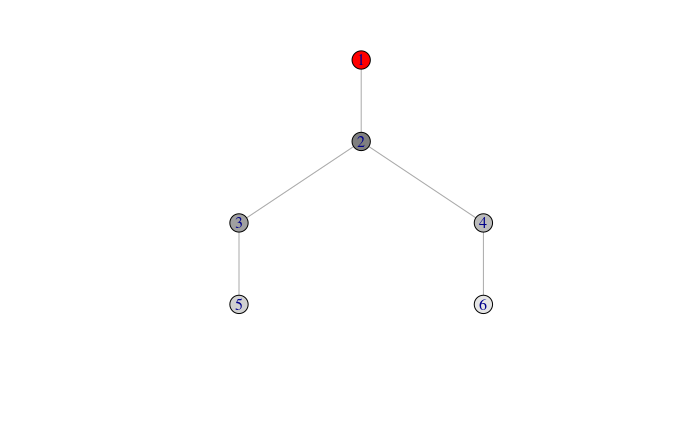This structure is can be used for any forum. Thus, given your favorite forum, you can start working with this package as soon as you are able to write your forum as a list of parent vectors.

The basic structure is therefore a parents vector. However, our current models convert these vectors into dataframes with some information that can be easily deduced from the parents vector and which will be needed by our algoritms. For instance, our parent vector from above will be transformed in a dataframe doing

# Vector representation
parents   <- c(1,2,2,3,4)
# Dataframe representation. Easy to make computations
df.thread

Where:

* post:           id of the node
* t:              time steps in which the node appeared (root appears at t=0)
* parent:         id of parent node
* popularity:     degree of parent node just before this node appeared
* root:           1 if the parent node is the root
* lag (recency):  time steps ellapsed since the parent post appeared.

### A real conversation

For Reddit forums, monthly dumps (in json format) can be found at:

And here is a code to parse one such monthly dumps and save it in a MySQL database:

We have already prepared some data from Reddit in the form of dataframes. We can load it, convert it to parent vectors, and plot it:

data("df.posts.france")
# Create dataframe structure dropping short threads
group_by(thread) %>% arrange(date) %>% filter(n()>10) %>%
mutate(pi = as.integer(match(parent, unique(parent))-1)) %>%
ungroup %>%
# Convert dataframe to list of parent vectors
do(thread=.$pi) %>% ungroup() %>% lapply(function(x) {(x)})  |=============================================================== | 95% ~0 s remaining |================================================================= | 98% ~0 s remaining  parents <- parents[] cat('Threads:', length(parents)) Threads: 17030 # Plot some threads in tree and graph representations par(mfrow = c(3,3)) for(i in 1:3){ plot.tree(parents[[i]]) }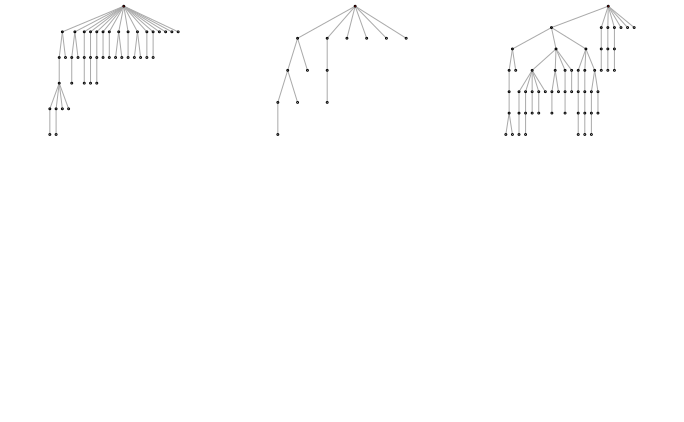# Inside the package Our conversation model is as follows: $p(\pi_t) \propto \alpha d_{k,t} + \beta_{k} + \tau^{t-k+1}$ In our package, we need: • This likelihood function.  likelihoods.R -> likelihood_post_Gomez2013(df.trees, alpha, beta, tau) • An estimation function based upon the likelihood:  estimators.R -> estimation_Gomez2013(df.trees, params){ (optimizes objective function likelihood_post_Gomez2013) • A function to generate synthetic data:  thread_generators.R -> gen.parentsvector.Gomez2013(n, alpha, beta, tau) (generates artificial parent vectors) Other useful files: • data_structures.R: functions to convert from parent vectors to dataframes and vice versa. • structural_properties.R: functions to compute structural properties of a forum dataset (synthetic or real) • plot_utils.R: functions to plot trees, etc. ### Generating synthetic conversations with a growth model (2 mins) We can chose some parameters for the model and generate artificial threads: alpha <- 0.5 beta <- 1 tau <- 0.8 ntrees <- 500 n = 100 parents <- replicate(ntrees, gen.parentsvector.Gomez2013(n, alpha, beta, tau), simplify = FALSE) par(mfrow = c(3,3)) for(i in 1:9){ plot.tree(parents[[i]]) }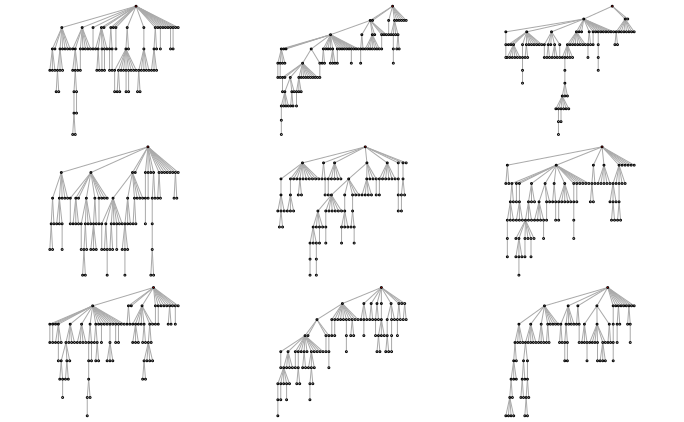## Sanity check (5 mins) Before fitting our model to real data, we will perform a sanity check so see whether we can recover the parameters of ou synthetic trees. First, let us see whether, the likelihood function for a given parameter given the others peaks around the true value: For alpha: par(mfrow = c(1,1)) df.trees <- all_parents_to_dataframe(parents) alpha_grid <- seq(0.1,5, by = 0.05) like <- rep(NA, length(alpha_grid)) for(i in 1:length(alpha_grid)){ like[i] <- likelihood_Gomez2013(df.trees, alpha_grid[i], beta, tau) } plot(alpha_grid, like, xlab = 'alpha') abline(v=alpha, col = 'blue')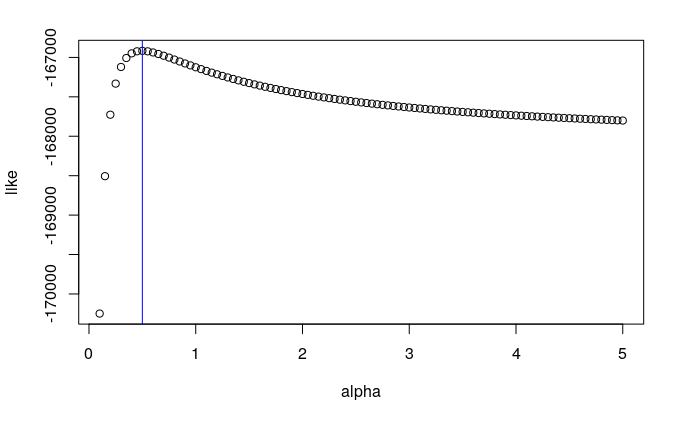For beta: beta_grid <- seq(0,2, by = 0.1) like <- rep(NA, length(beta_grid)) for(i in 1:length(beta_grid)){ like[i] <- likelihood_Gomez2013(df.trees, alpha=alpha, beta_grid[i], tau) } plot(beta_grid, like, xlab = 'beta') abline(v=beta, col = 'blue')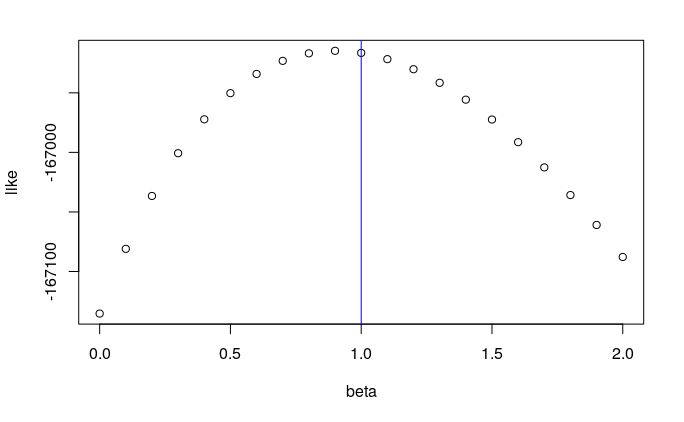For tau: tau_grid <- seq(0,1, by = 0.05) like <- rep(NA, length(tau_grid)) for(i in 1:length(tau_grid)){ like[i] <- likelihood_Gomez2013(df.trees, alpha, beta, tau_grid[i]) } plot(tau_grid, like, xlab = 'tau') abline(v=tau, col = 'blue')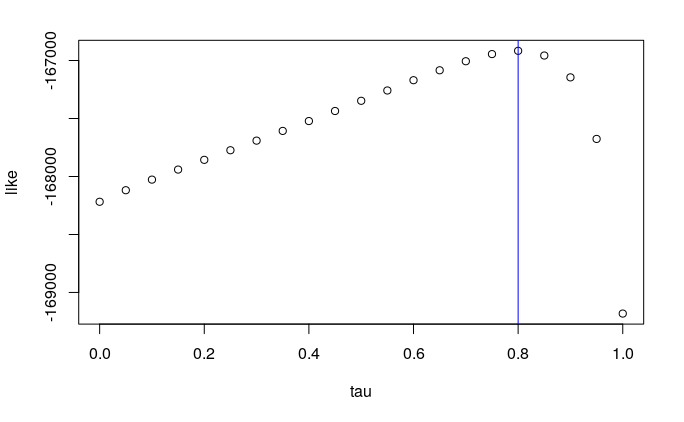Now, we can check whether, for different parameter initializations, we get find the true parameters: alpha <- 0.5 beta <- 1 tau <- 0.8 ntrees <- 500 n = 100 df.trees <- all_parents_to_dataframe(parents) df.results <- data.frame() for(ntrees in c(10, 1000)){ # Generate trees parents <- replicate(ntrees, gen.parentsvector.Gomez2013(n, alpha, beta, tau), simplify = FALSE) df.trees <- all_parents_to_dataframe(parents) # Estimate with different init parameters for(xp in 1:10){ alpha_0 <- runif(1) beta_0 <- runif(1)*10 tau_0 <- runif(1, max=0.99) res <- estimation_Gomez2013(df.trees = df.trees, params=list(alpha_0, beta_0, tau_0)) res$ntrees <- ntrees
df.results <- rbind(df.results, res)
}
}
library(tidyr)

Attaching package: ‘tidyr’

The following object is masked from ‘package:igraph’:

crossing
df.errors <- df.results
df.errors$alpha <- df.errors$alpha - alpha
df.errors$beta <- df.errors$beta   - beta
df.errors$tau <- df.errors$tau     - tau
df.errors <- gather(df.errors, param, value, -likelihood, -ntrees)
df.errors$param <- factor(df.errors$param, levels = c("beta", "alpha", "tau"))
ggplot(df.errors, aes(x=param, y= value)) +
geom_point() +
#geom_boxplot() + ylim(-5,5) +
facet_grid(.~ntrees) + theme_bw()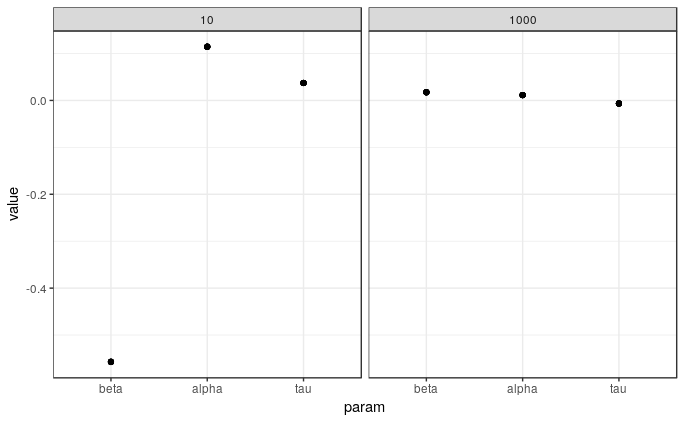## Find model parameters that fit real conversations (2 mins)

Now we the parameters to the real threads.

# Load again the real data
data("df.posts.france")
do(thread=.$pi) %>% ungroup() %>% lapply(function(x) {(x)}) parents <- parents[] cat('Threads:', length(parents)) Threads: 17030 # Estimate parameters ---------------------------------------------------------- # Store in dataframe format. # Each line contains the post id, the chosen parent # and the features of its parent (popularity, lag, root) at the # moment (t) of that choice. df.trees <- all_parents_to_dataframe(parents) # Estimate alpha, beta, tau parameters res <- estimation_Gomez2013(df.trees = df.trees, params=list(alpha=0.5, beta=0.6, tau=0.5)) res $alpha
$beta  3.182121$tau
$likelihood  -3103075 ## Compare structural properties (5 mins) Finally, we check whether our model reproduces the structural properties of the real data. # Generate threads with the estimated parameters sizes <- sapply(parents, function(x) length(x)) parents_hat <- list() for(i in 1:length(sizes)){ parents_hat[[i]] <- gen.parentsvector.Gomez2013(sizes[i], res$alpha, res$beta, res$tau)
}